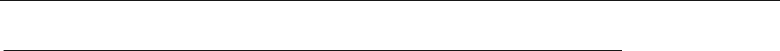Textbook Notes (290,000)
CA (170,000)
UTSC (20,000)
Psychology (10,000)
PSYB01H3 (600)
Chapter 12

# PSYB01H3 Chapter Notes - Chapter 12: Frequency Distribution, Dependent And Independent Variables, Level Of Measurement

Department
Psychology
Course Code
PSYB01H3
Professor
Connie Boudens
Chapter
12

This preview shows page 1. to view the full 5 pages of the document.Chapter 12 - Understanding research results: description and correlation
Stats are used for describing data and making inferences on sample data
Scales of Measurement: a review
Levels of a variable can be decribed by 4 scales
oNominal: no numerical, quantitative properties
oOrdinal: rank order
oInterval: quantitative properties, no absolute 0
oRatio: quantitative properties, has an absolute 0
Analyzing the results if research investigation
Depending on the way the variables are studied there are 3 basic ways of describing the results
oComparing group percentages
oCorrelating scores of individuals on two variables
oComparing group means
Comparing group percentages
Example: studying if which females or males like traveling more
oLets say out of 50 females and 50 males
80% of females like to travel
60% of males like to travel
oNeed to focus on % because of variable of liking travel or disliking travel is nominal
Correlating individual scores
Used when you do not have distinct subject groups
Individuals are measured on 2 variables
oEach variable has a range of numerical values
Comparing group means
Example: studying effect of exposure to an aggressive adult on children's play
oOn average how many aggressive acts did the children perform during play in both
groups?
Aggression is a ratio scale variable because there is a true zero and equal intervals
Frequency distributions
When analyzing results it is useful to start by making a frequency distribution of the data
Frequency Distribution: indicates the number of individuals that receive each possible score on a
variable
oUseful to look at this in terms of %s
Example: how many students in a class received a specific score on an exam
Graphing frequency Distributions
Pie Charts: divide a whole circle or pie into slices that represent relative percentages
oUseful when representing nominal scale information

Unlock to view full version

Only page 1 are available for preview. Some parts have been intentionally blurred.

Bar graphs: use a separate and distinct bar for each piece of information
oX-axis is the independent variable
oy-axis is the dependent variable
Frequency polygons: use a line to represent frequencies
oUseful when representing interval or ratio scales
oCan have more then one line to represent more then one group
Histograms: uses bars to display a frequency distribution for a quantitative variable
oScale values are continuous and show increasing amounts on a variable such as age,
blood pressure or stress
oBars are drawn next to each other since values are continuous
Looking at frequency distributions allows you to directly observe how your participants
responded
oCan look at what scores are most frequent and the shape of the distribution of scores
oFind "outliers" or unusual, unexpected scores
Descriptive statistics
Descriptive statistics allow researchers to make precise statements about the data
oTwo statistics are needed to describe the data
oOne number can be used to describe the central tendency or how participants scored
overall while another number describes the variability or how widely the distribution of
These 2 numbers summarize the information contained in a frequency distribution
Central tendency
Central tendency: a central tendency statistic tells us what the sample as a whole or on the
average is like
oThree measures of central tendency:
Mean: the average
Abbreviated as "M"
Indicator of central tendency only when scores are measured on an
interval or ratio scale
Median: the score that divides the group in half
Abbreviated as "Mdn"
Indicator of central tendency only when scores are measured on an ordinal
scale
Also useful with interval and ratio scale variables
Mode: the most frequent score
Only measure of central tendency that is appropriate if a nominal scale is
used
oThe median or mode can be a better indicator of central tendency than the mean if a few
unusual scores bias the mean
Variability
A measure of variability is a number that characterizes the amount of spread in a distribution of
scores